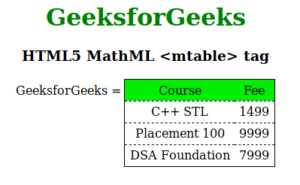# HTML5 | MathML <mtable> Tag

• Last Updated : 22 Aug, 2021

The MathML <mtable> tag is used to create table or the matrices in HTML5. This tag is similar to the normal HTML <table> tag. The <table> has the <tr>, <td> and <td> tag, similarly it has the <mtr>, <mtd> and <mtd> tag, that act as same as those. But we don’t use the <table> and other sub tags to create the matrices. Here <mtable> is useful to create matrices. Because the MathML is all about the mathematical expression representation in HTML.

Syntax:

`<mtable attributes="value"> child elements </mtable>`

Attributes: It accepts some attributes which are listed below:

• align: This attribute holds the alignment of the tables. Possible values are axis, baseline, bottom, center and top.
• class|id|style: This attribute is used to hold the styles of the child elements.
• columnalign: This attribute holds the horizontal alignment of the table cells. Possible values are left, right and center.
• columnlines: This attribute holds the border style value for the column-lines. Possible values are none, solid and dashed.
• displaystyle: It is a Boolean value attribute that defines whether more vertical space is used for displayed equations or more compact layout is used to display formulas.
• frame: This attribute holds the border value for the entire table. Possible values are none, solid and dashed.
• framespacing: This attribute defines the space between the table and the frame.
• href: This attribute is used to hold any hyperlink to a specified URL.
• mathbackground: This attribute holds the value of the math expressions background color.
• mathcolor: This attribute holds the color of the math expressions.
• rowalign: This attribute holds vertical alignment of the table cells. Possible values are top, bottom and center.
• rowlines: This attribute holds the row borders value that multiple value can be separated by using a space can consider also. Possible values are none, solid and dashed.
• width: This attribute holds the width value length.

Note: There are few more attribute but those are not implemented yet like rowspacing, side, minlabelspacing, groupalign etc.
Below example illustrates the MathML <mtable> tag in HTML5:

Example:

## html

 ```<``html``>` `<``head``>``    ``<``title``>HTML5 MathML mtable tag``` `<``body``>``    ``<``center``>``        ``<``h1` `style``=``"color:green"``>``            ``GeeksforGeeks``        ``` `        ``<``h3``>HTML5 MathML <``mtable``> tag` `        ``<``math``>``            ``<``mi``>GeeksforGeeks``            ``<``mo``>=``            ``<``mtable` `frame``=``"solid"` `rowlines``=``"dashed"` `align``=``"axis 1"``>``                ``<``mtr` `mathbackground``=``"green;"``>``                    ``<``mtd``>Course``                    ``<``mtd``>Fee``                ````                ``<``mtr``>``                    ``<``mtd``>``                        ``<``mi``>C++ STL``                    ````                    ``<``mtd``>``                        ``<``mi``> 1499``                    ````                ````                ``<``mtr``>``                    ``<``mtd``>``                        ``<``mi``>Placement 100 ``                    ````                    ``<``mtd``>``                        ``<``mi``>9999 ``                    ````                ````                ``<``mtr``>``                    ``<``mtd``>``                        ``<``mi``>DSA Foundation ``                    ````                    ``<``mtd``>``                        ``<``mi``>7999``                    ````                ````            ````        ````    ````` ``

Output:Supported Browsers: The browsers supported by HTML5 MathML <mtable> tag are listed below:

• Firefox
• Safari

My Personal Notes arrow_drop_up